# Domains and Ranges of Standard Functions Set 2

Go back to  'Functions'

6. Reciprocal function \begin{align}f\,(x) = \frac{1}{x}\end{align}

Domain = $$\mathbb{R}$$ – {0}

Range = $$\mathbb{R}$$– {0}

{Range is $$\mathbb{R}$$ – {0} because for no value of x is f (x) = 0}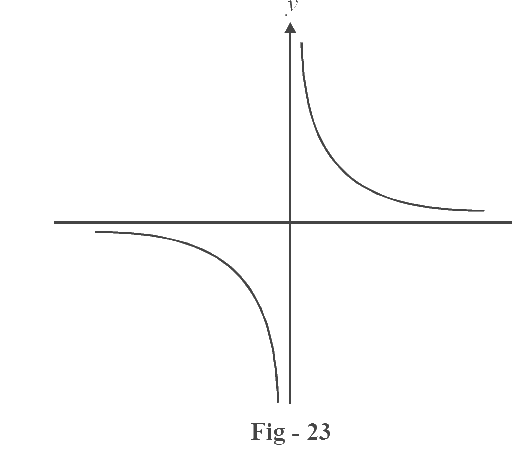7. Step function  $$f\,(x)\, = \,0\;\; if \;x < 0$$

$$1\;\; if\; x \geqslant 0$$

Domain = $$\mathbb{R}$$

Range = {0,1}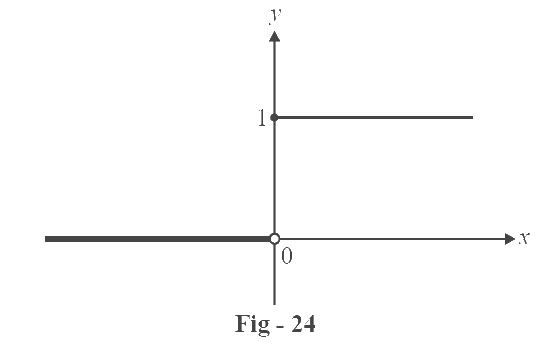8. Modulus function  $$f\left( x \right) = \left| x \right|\, = \,\,\,x\,\,\,\,if\,\,x \ge \;0$$

$$- x\,\;\,if\;x\; < \;0$$

Basically, this function gives the magnitude of a number and strips it off its negative sign,if it is negative.

Domain=$$\mathbb{R}$$

Range = [0, $$\infty$$)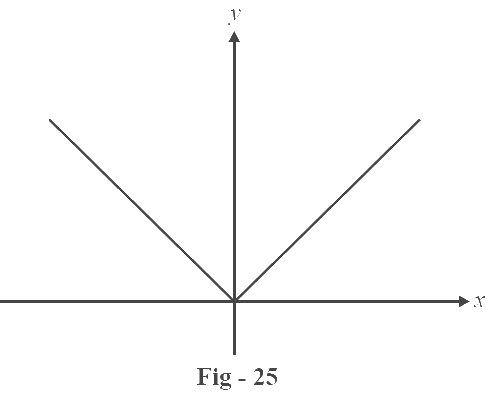9. Greatest integer function $$f\,(x)=[x]$$

This is an interesting function. It is defined as the largest integer less than or equal to $$x$$.

For example, [1.3] = 1        [–1.3] = –2         [0.6] = 0    [$$\pi$$] =3   etc.

To draw its graph, we note that $$f\,(x)=0$$ for $$0 \le x < 1$$, $$f\,(x)=1$$ for $$1 \le x < 2$$, $$f\,(x)=-1$$ for  $$- 1 \le x < 0$$ and so on.

Therefore, if $$x$$ lies in the interval $$[n, n + 1)$$, then the value of $$[x]$$ is $$n$$.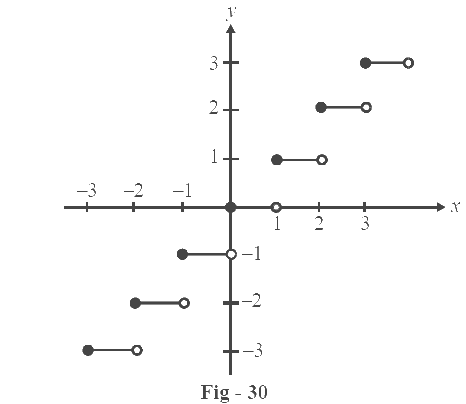Domain = $$\mathbb{R}$$

Range = $$\mathbb{Z}$$ (set of all integers)

Properties

(i)  $$[x + n] = [x] + n,n \in \mathbb{Z}$$

(ii)  $$[ - x] = - [x] - 1,\;x \notin\mathbb{Z}$$

$$[ - x] = - [x],\;\;\;\;\;\;\;\;x \in \mathbb{Z}$$

Verify these properties carefully because they are very important

10. Fractional part f (x) = {x}

Every number x can be written as the sum of its integer and fractional parts. So, fractional part

{x} = x – [x]

or in other words, fractional part of a number is the difference between that number and its integral part [x]

We example, {1.3}= 0.3, {-1.3} = 0.7 and so on.

We can obviously see that $$0 \le \{ x\} < 1$$

To draw the graph, we note that f (x) = x for $$0\underline < x < 1$$ because in this interval, [x] = 0. As soon as x becomes 1, f (x­­­­­­­­) drops again to 0, and then starts increasing as x increases. And so on, we see that at each integral value of x, f (x) becomes 0, then increases and approaches 1 as x approaches the next integer, and finally drops again to 0, never becoming 1.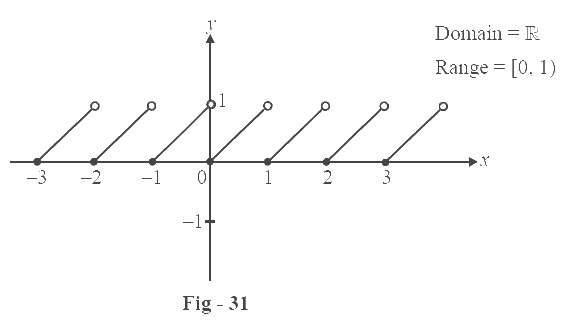Properties

(i) $$\left\{ {x + n} \right\} = \left\{ x \right\},n \in \mathbb{Z}$$

(ii)$$\left\{ { - x} \right\} = 1 - \left\{ x \right\},n \in \mathbb{Z}$$

$$\left\{ { - x} \right\} = 0,\;\;\;n \in \mathbb{Z}$$